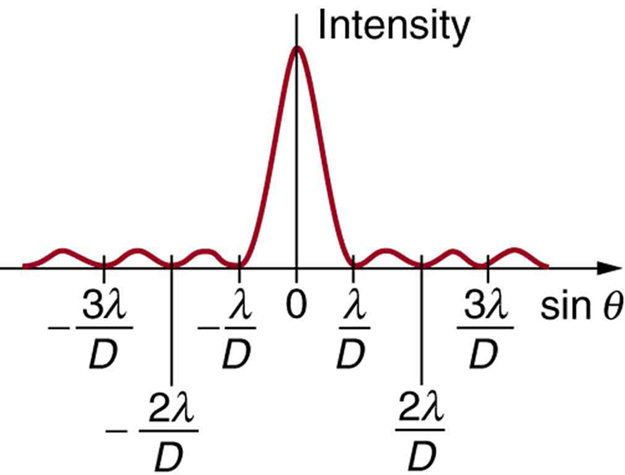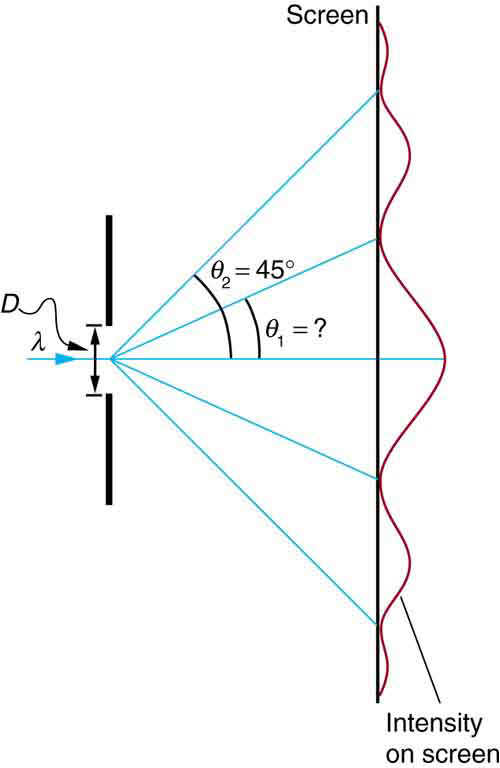# 27.5 Single slit diffraction  (Page 2/4)

 Page 2 / 4A graph of single slit diffraction intensity showing the central maximum to be wider and much more intense than those to the sides. In fact the central maximum is six times higher than shown here.

Thus, to obtain destructive interference for a single slit    ,

$D\text{sin}\phantom{\rule{0.25em}{0ex}}\theta =\mathrm{m\lambda },\phantom{\rule{0.25em}{0ex}}\text{for}\phantom{\rule{0.25em}{0ex}}m=\text{1,}\phantom{\rule{0.25em}{0ex}}\text{–1,}\phantom{\rule{0.25em}{0ex}}\text{2,}\phantom{\rule{0.25em}{0ex}}\text{–2,}\phantom{\rule{0.25em}{0ex}}\text{3,}\phantom{\rule{0.25em}{0ex}}\dots \phantom{\rule{0.25em}{0ex}}\text{(destructive),}$

where $D$ is the slit width, $\lambda$ is the light’s wavelength, $\theta$ is the angle relative to the original direction of the light, and $m$ is the order of the minimum. [link] shows a graph of intensity for single slit interference, and it is apparent that the maxima on either side of the central maximum are much less intense and not as wide. This is consistent with the illustration in [link] (b).

## Calculating single slit diffraction

Visible light of wavelength 550 nm falls on a single slit and produces its second diffraction minimum at an angle of $\text{45.0º}$ relative to the incident direction of the light. (a) What is the width of the slit? (b) At what angle is the first minimum produced?A graph of the single slit diffraction pattern is analyzed in this example.

Strategy

From the given information, and assuming the screen is far away from the slit, we can use the equation $D\text{sin}\phantom{\rule{0.25em}{0ex}}\theta =\text{mλ}$ first to find $D$ , and again to find the angle for the first minimum ${\theta }_{1}$ .

Solution for (a)

We are given that $\lambda =\text{550 nm}$ , $m=2$ , and ${\theta }_{2}=\text{45.0º}$ . Solving the equation $D\phantom{\rule{0.25em}{0ex}}\text{sin}\phantom{\rule{0.25em}{0ex}}\theta =\text{mλ}$ for $D$ and substituting known values gives

$\begin{array}{lll}D& =& \frac{\mathrm{m\lambda }}{\phantom{\rule{0.25em}{0ex}}\text{sin}\phantom{\rule{0.25em}{0ex}}{\theta }_{2}}=\frac{2\left(\text{550 nm}\right)}{\text{sin 45.0º}}\\ & =& \frac{\text{1100}×{\text{10}}^{-9}}{0.707}\\ & =& 1.56×{\text{10}}^{-6}.\end{array}$

Solution for (b)

Solving the equation $D\phantom{\rule{0.25em}{0ex}}\text{sin}\phantom{\rule{0.25em}{0ex}}\theta =\text{mλ}$ for $\text{sin}\phantom{\rule{0.25em}{0ex}}{\theta }_{1}$ and substituting the known values gives

$\text{sin}\phantom{\rule{0.25em}{0ex}}{\theta }_{1}=\frac{\mathrm{m\lambda }}{D}=\frac{1\left(\text{550}×{\text{10}}^{-9}\phantom{\rule{0.25em}{0ex}}\text{m}\right)}{1\text{.}\text{56}×{\text{10}}^{-6}\phantom{\rule{0.25em}{0ex}}\text{m}}\text{.}$

Thus the angle ${\theta }_{1}$ is

${\theta }_{1}={\text{sin}}^{-1}\phantom{\rule{0.25em}{0ex}}\text{0.354}=\text{20.7º.}$

Discussion

We see that the slit is narrow (it is only a few times greater than the wavelength of light). This is consistent with the fact that light must interact with an object comparable in size to its wavelength in order to exhibit significant wave effects such as this single slit diffraction pattern. We also see that the central maximum extends $\text{20.7º}$ on either side of the original beam, for a width of about $\text{41º}\phantom{\rule{0.25em}{0ex}}$ . The angle between the first and second minima is only about $\text{24º}\phantom{\rule{0.25em}{0ex}}\left(\text{45.0º}-20.7º\right)$ . Thus the second maximum is only about half as wide as the central maximum.

## Section summary

• A single slit produces an interference pattern characterized by a broad central maximum with narrower and dimmer maxima to the sides.
• There is destructive interference for a single slit when $D\phantom{\rule{0.25em}{0ex}}\text{sin}\phantom{\rule{0.25em}{0ex}}\theta =\text{mλ},\phantom{\rule{0.25em}{0ex}}\text{(for}\phantom{\rule{0.25em}{0ex}}m=\text{1,}\phantom{\rule{0.25em}{0ex}}\text{–1,}\phantom{\rule{0.25em}{0ex}}\text{2,}\phantom{\rule{0.25em}{0ex}}\text{–2,}\phantom{\rule{0.25em}{0ex}}\text{3,}\phantom{\rule{0.25em}{0ex}}\dots \right)$ , where $D$ is the slit width, $\lambda$ is the light’s wavelength, $\theta$ is the angle relative to the original direction of the light, and $m$ is the order of the minimum. Note that there is no $m=0$ minimum.

## Conceptual questions

As the width of the slit producing a single-slit diffraction pattern is reduced, how will the diffraction pattern produced change?

## Problems&Exercises

(a) At what angle is the first minimum for 550-nm light falling on a single slit of width $1\text{.}\text{00}\phantom{\rule{0.25em}{0ex}}\text{μm}$ ? (b) Will there be a second minimum?

(a) $\text{33}\text{.}4º$

(b) No

(a) Calculate the angle at which a $2\text{.}\text{00}\text{-μm}$ -wide slit produces its first minimum for 410-nm violet light. (b) Where is the first minimum for 700-nm red light?

what is thermodynamics
thermodynamic is a branch of physics that teaches on the relationship about heat and anyother form of energy
Emmanuel
if l cary box and stop is ther any work
no that because u have moved no distance. for work to be performed a force needs to be applied and a distance needs to be moved
Emmanuel
Different between fundamental unit and derived unit
fundamental unit are independent quantities that do not depend on any other unit while derived unit are quantities that depend on two or more units for it definition
Emmanuel
what is nuclear fission
hello
Shawty
are you there
Shawty
Shawty
what is a vector
vectors are quantities that have numerical value or magnitude and direction.
what is regelation
vector is any quantity that has magnitude and direction
Emmanuel
Physics is a physical science that deals with the study of matter in relation to energy
Hi
Jimoh
hello
Salaudeen
hello
Yes
Maxamuud
hi everyone
what is physics
physics is a physical science that deals with the study of matter in relation to energy
Osayuwa
a15kg powerexerted by the foresafter 3second
what is displacement
movement in a direction
Jason
hello
Hosea
Hey
Smart
haider
Explain why magnetic damping might not be effective on an object made of several thin conducting layers separated by insulation? can someone please explain this i need it for my final exam
Hi
saeid
hi
Yimam
Hi
Jimoh
An object made of several thin conducting layers separated by insulation may not be affected by magnetic damping because the eddy current produced in each layer due to induction will be very small and the opposing magnetic flux produced by the eddy currents will be very small
What is thê principle behind movement of thê taps control
while
Hosea
what is atomic mass
this is the mass of an atom of an element in ratio with the mass of carbon-atom
Chukwuka
show me how to get the accuracies of the values of the resistors for the two circuits i.e for series and parallel sides
Explain why it is difficult to have an ideal machine in real life situations.
tell me
Promise
what's the s . i unit for couple?
Promise
its s.i unit is Nm
Covenant
Force×perpendicular distance N×m=Nm
Oluwakayode
İt iş diffucult to have idêal machine because of FRİCTİON definitely reduce thê efficiency
Oluwakayode
It is difficult to have an ideal machine in real life situation because in ideal machines all the input energy should be converted to output energy . But , some part of energy is always lost in overcoming friction and input energy is always greater than output energy . Hence , no machine is ideal.
if the classica theory of specific heat is valid,what would be the thermal energy of one kmol of copper at the debye temperature (for copper is 340k)

#### Get Jobilize Job Search Mobile App in your pocket Now!ByByBy Janet ForresterBy Frank LevyBy Janet ForresterBy OpenStaxBy Janet ForresterBy OpenStaxBy OpenStaxBy Sebastian Sieczko...By Megan EarhartBy Brooke Delaney